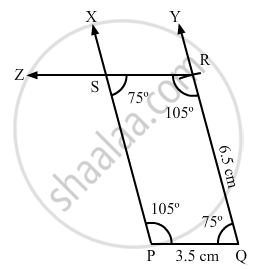Share

# Construct a Quadrilateral Pqrs, Where Pq = 3.5 Cm, Qr = 6.5 Cm, ∠P = ∠R = 105° and ∠S = 75°. - Mathematics

Course
ConceptConstructing a Quadrilateral When Two Adjacent Sides and Three Angles Are Known

#### Question

Construct a quadrilateral PQRS, where PQ = 3.5 cm, QR = 6.5 cm, ∠P = ∠R = 105° and ∠S = 75°.

#### Solution

We know that the sum of all the angles in a quadrilateral is 360 .

i . e . , ∠P + ∠Q + ∠R + ∠S = 360°

⇒ ∠Q = 75°

Steps of construction:

Step I: Draw PQ = 3 . 5 cm .

Step II: Construct ∠XPQ = 105° at P and ∠PQY = 75° at Q .

Step III: With Q as the centre and radius 6 . 5 cm, cut off QR = 6 . 5

Step IV: At R, draw ∠QRZ = 105° such that it meets PX at S .